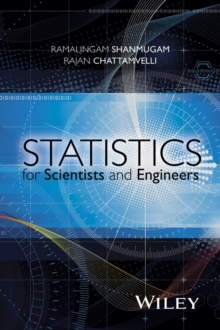Supporting your high street Find out how »
• My Account# Statistics for Scientists and Engineers Hardback

#### Description

This book provides the theoretical framework needed to build, analyze and interpret various statistical models.

It helps readers choose the correct model, distinguish among various choices that best captures the data, or solve the problem at hand.

This is an introductory textbook on probability and statistics.

The authors explain theoretical concepts in a step-by-step manner and provide practical examples.

The introductory chapter in this book presents the basic concepts.

Next, the authors discuss the measures of location, popular measures of spread, and measures of skewness and kurtosis.

Probability theory, discrete distributions, and important continuous distributions that are often encountered in practical applications are analyzed.

Mathematical Expectation is covered, along with Generating Functions and Functions of Random Variables.

It discusses joint distributions, and novel methods to find the mean deviation of discrete and continuous statistical distributions. * Provides insight on coding complex algorithms using the 'loop unrolling technique' * Covers illuminating discussions on Poisson limit theorem, central limit theorem, mean deviation generating functions, CDF generating function and extensive summary tables * Contains extensive exercises at the end of each chapter and examples from interdisciplinary fields Statistics for Scientists and Engineers is a great resource for students in engineering, physical sciences, and management, and also practicing engineers who require skill sets to model practical problems in a statistical setting.

#### Information

• Format: Hardback
• Pages: 496 pages
• Publisher: John Wiley & Sons Inc
• Publication Date:
• Category: Probability & statistics
• ISBN: 9781118228968

£110.00

£97.89

on all orders

###### Pick up orders

from local bookshops

£46.95

£46.75

£67.95

£67.69